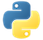# CombineGloballyPydoc

Combines all elements in a collection.

## Examples

In the following examples, we create a pipeline with a `PCollection` of produce. Then, we apply `CombineGlobally` in multiple ways to combine all the elements in the `PCollection`.

`CombineGlobally` accepts a function that takes an `iterable` of elements as an input, and combines them to return a single element.

### Example 1: Combining with a function

We define a function `get_common_items` which takes an `iterable` of sets as an input, and calculates the intersection (common items) of those sets.

``````import apache_beam as beam

def get_common_items(sets):
# set.intersection() takes multiple sets as separete arguments.
# We unpack the `sets` list into multiple arguments with the * operator.
# The combine transform might give us an empty list of `sets`,
# so we use a list with an empty set as a default value.
return set.intersection(*(sets or [set()]))

with beam.Pipeline() as pipeline:
common_items = (
pipeline
| 'Create produce' >> beam.Create([
{'🍓', '🥕', '🍌', '🍅', '🌶️'},
{'🍇', '🥕', '🥝', '🍅', '🥔'},
{'🍉', '🥕', '🍆', '🍅', '🍍'},
{'🥑', '🥕', '🌽', '🍅', '🥥'},
])
| 'Get common items' >> beam.CombineGlobally(get_common_items)
| beam.Map(print))``````

Output:

``{'🍅', '🥕'}``View source code

### Example 2: Combining with a lambda function

We can also use lambda functions to simplify Example 1.

``````import apache_beam as beam

with beam.Pipeline() as pipeline:
common_items = (
pipeline
| 'Create produce' >> beam.Create([
{'🍓', '🥕', '🍌', '🍅', '🌶️'},
{'🍇', '🥕', '🥝', '🍅', '🥔'},
{'🍉', '🥕', '🍆', '🍅', '🍍'},
{'🥑', '🥕', '🌽', '🍅', '🥥'},
])
| 'Get common items' >>
beam.CombineGlobally(lambda sets: set.intersection(*(sets or [set()])))
| beam.Map(print))``````

Output:

``{'🍅', '🥕'}``View source code

### Example 3: Combining with multiple arguments

You can pass functions with multiple arguments to `CombineGlobally`. They are passed as additional positional arguments or keyword arguments to the function.

In this example, the lambda function takes `sets` and `exclude` as arguments.

``````import apache_beam as beam

with beam.Pipeline() as pipeline:
common_items_with_exceptions = (
pipeline
| 'Create produce' >> beam.Create([
{'🍓', '🥕', '🍌', '🍅', '🌶️'},
{'🍇', '🥕', '🥝', '🍅', '🥔'},
{'🍉', '🥕', '🍆', '🍅', '🍍'},
{'🥑', '🥕', '🌽', '🍅', '🥥'},
])
| 'Get common items with exceptions' >> beam.CombineGlobally(
lambda sets, exclude: \
set.intersection(*(sets or [set()])) - exclude,
exclude={'🥕'})
| beam.Map(print)
)``````

Output:

``{'🍅'}``View source code

### Example 4: Combining with side inputs as singletons

If the `PCollection` has a single value, such as the average from another computation, passing the `PCollection` as a singleton accesses that value.

In this example, we pass a `PCollection` the value `'🥕'` as a singleton. We then use that value to exclude specific items.

``````import apache_beam as beam

with beam.Pipeline() as pipeline:
single_exclude = pipeline | 'Create single_exclude' >> beam.Create(['🥕'])

common_items_with_exceptions = (
pipeline
| 'Create produce' >> beam.Create([
{'🍓', '🥕', '🍌', '🍅', '🌶️'},
{'🍇', '🥕', '🥝', '🍅', '🥔'},
{'🍉', '🥕', '🍆', '🍅', '🍍'},
{'🥑', '🥕', '🌽', '🍅', '🥥'},
])
| 'Get common items with exceptions' >> beam.CombineGlobally(
lambda sets, single_exclude: \
set.intersection(*(sets or [set()])) - {single_exclude},
single_exclude=beam.pvalue.AsSingleton(single_exclude))
| beam.Map(print)
)``````

Output:

``{'🍅'}``View source code

### Example 5: Combining with side inputs as iterators

If the `PCollection` has multiple values, pass the `PCollection` as an iterator. This accesses elements lazily as they are needed, so it is possible to iterate over large `PCollection`s that won’t fit into memory.

``````import apache_beam as beam

with beam.Pipeline() as pipeline:
exclude = pipeline | 'Create exclude' >> beam.Create(['🥕'])

common_items_with_exceptions = (
pipeline
| 'Create produce' >> beam.Create([
{'🍓', '🥕', '🍌', '🍅', '🌶️'},
{'🍇', '🥕', '🥝', '🍅', '🥔'},
{'🍉', '🥕', '🍆', '🍅', '🍍'},
{'🥑', '🥕', '🌽', '🍅', '🥥'},
])
| 'Get common items with exceptions' >> beam.CombineGlobally(
lambda sets, exclude: \
set.intersection(*(sets or [set()])) - set(exclude),
exclude=beam.pvalue.AsIter(exclude))
| beam.Map(print)
)``````

Output:

``{'🍅'}``View source code

Note: You can pass the `PCollection` as a list with `beam.pvalue.AsList(pcollection)`, but this requires that all the elements fit into memory.

### Example 6: Combining with side inputs as dictionaries

If a `PCollection` is small enough to fit into memory, then that `PCollection` can be passed as a dictionary. Each element must be a `(key, value)` pair. Note that all the elements of the `PCollection` must fit into memory for this. If the `PCollection` won’t fit into memory, use `beam.pvalue.AsIter(pcollection)` instead.

``````import apache_beam as beam

def get_custom_common_items(sets, options):
sets = sets or [set()]
common_items = set.intersection(*sets)
common_items |= options['include']  # union
common_items &= options['exclude']  # intersection
return common_items

with beam.Pipeline() as pipeline:
options = pipeline | 'Create options' >> beam.Create([
('exclude', {'🥕'}),
('include', {'🍇', '🌽'}),
])

custom_common_items = (
pipeline
| 'Create produce' >> beam.Create([
{'🍓', '🥕', '🍌', '🍅', '🌶️'},
{'🍇', '🥕', '🥝', '🍅', '🥔'},
{'🍉', '🥕', '🍆', '🍅', '🍍'},
{'🥑', '🥕', '🌽', '🍅', '🥥'},
])
| 'Get common items' >> beam.CombineGlobally(
get_custom_common_items, options=beam.pvalue.AsDict(options))
| beam.Map(print))``````

Output:

``{'🍅', '🍇', '🌽'}``View source code

### Example 7: Combining with a `CombineFn`

The more general way to combine elements, and the most flexible, is with a class that inherits from `CombineFn`.

``````import apache_beam as beam

class PercentagesFn(beam.CombineFn):
def create_accumulator(self):
return {}

# accumulator == {}
# input == '🥕'
if input not in accumulator:
accumulator[input] = 0  # {'🥕': 0}
accumulator[input] += 1  # {'🥕': 1}
return accumulator

def merge_accumulators(self, accumulators):
# accumulators == [
#     {'🥕': 1, '🍅': 2},
#     {'🥕': 1, '🍅': 1, '🍆': 1},
#     {'🥕': 1, '🍅': 3},
# ]
merged = {}
for accum in accumulators:
for item, count in accum.items():
if item not in merged:
merged[item] = 0
merged[item] += count
# merged == {'🥕': 3, '🍅': 6, '🍆': 1}
return merged

def extract_output(self, accumulator):
# accumulator == {'🥕': 3, '🍅': 6, '🍆': 1}
total = sum(accumulator.values())  # 10
percentages = {item: count / total for item, count in accumulator.items()}
# percentages == {'🥕': 0.3, '🍅': 0.6, '🍆': 0.1}
return percentages

with beam.Pipeline() as pipeline:
percentages = (
pipeline
| 'Create produce' >> beam.Create(
['🥕', '🍅', '🍅', '🥕', '🍆', '🍅', '🍅', '🍅', '🥕', '🍅'])
| 'Get percentages' >> beam.CombineGlobally(PercentagesFn())
| beam.Map(print))``````

Output:

``{'🥕': 0.3, '🍅': 0.6, '🍆': 0.1}``View source code

You can use the following combiner transforms:Pydoc